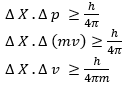# De-Broglie equation and Heisenberg Uncertainity Principle (chemistry)

## Dual Behaviour Of Matter (De-Broglie Equation)

According to this, matter like radiation posses dual behavior i.e. Wave nature as well as particle nature.
This means that photons, electrons possess both momentum as well as wavelength .
$\lambda =\frac {h}{mv}= \frac {h}{p}$
Here λ is wavelength , p is the momentum

## Solved Examples

Question 1
If velocity of electron in this microscope is 1.6 X 106 m/s. Calculate the de-Broglie wavelength.
Solution
Given:- 1.6 X 106 m/s.
m = 9.1 X 10-31 kg
h = 6.6 X 10-34 J/s
λ=?
$\lambda =\frac {h}{mv}= \frac {h}{p}$
=6.6 X 10-34 /( 9.1 X 10-31 X 1.6 X 106 )
= 0.4 X 10-9 m
Question 2
The velocity associated with proton moving in a potential difference of 1000 V is 4.37 X 105 m/s. If the hockey ball of mass 0.1 kg is moving with this velocity; calculate λ the associated with this velocity.
Solution
m = 0.1kg = 100 gm
v = 4.37 X 105 m/s
h = 6.6 X 10-34 J/s
λ=?
$\lambda =\frac {h}{mv}= \frac {h}{p}$
=6.6 X 10-34 /(.1 X 4.37 X 105 )
=15.10 X10-39 m

## Relation between Circumference of Bohr orbit for Hydrogen atom and be de- Broglie Wavelength

According to Bohr theory
$(L) = n \frac {h}{2 \pi}$
where n = no. of shell
This can be written as
$mvr = n \frac {h}{2 \pi}$
$2 \pi r= n \frac {h}{mv}$
According to De- Broglie relation
$\lambda =\frac {h}{mv}= \frac {h}{p}$
So, 2π r = n λ

## Solved Examples

Question 1
If velocity of electron in this microscope is 2.19 X 106 m/s. Calculate the de-Broglie wavelength.
Solution
Given:- v=2.19 X 106 m/s.
m = 9.1 X 10-31 kg
h = 6.6 X 10-34 J/s
λ=?
$\lambda =\frac {h}{mv}= \frac {h}{p}$
=6.6 X 10-34 /( 9.1 X 10-31 X 2.19 X 106 )
= 0.3 X 10-9 m

## Heisenberg Uncertainty Principle

According to this, it is impossible to determine simultaneously the exact position & the exact momentum (or velocity) of an electron
Mathematically:ΔX is uncertainty in position Δ v is in velocity.
The effect of this principle is only significant for microscopic objects and it is negligibile for macroscopic objects
Question 1
If the position of electron is measured with accuracy of 0.002 nm. Calculate uncertainty in momentum of e
Solution
ΔX. Δ p = h/4π
$0.002 \times 10^(-9 ).\Delta p= \frac {6.6 \times 10^{-34}}{4 \times 3.14}$
$\Delta p= \frac {6.6 \times 10^{-34}}{4 \times 3.14 \times 0.002 \times 10^{-9} }$
= 2.637 X 10-23 kg m/s

### Significance of Uncertainty Principle:-

1. It rules out definite paths or orbits of electrons and other similar particles.
2. This principle is significant only for microscope object like electrons and negligible for macroscopic objects

### Reason for failure of Bohr model:-

1. The wave character is not considered in Bohr model.
2. According to Bohr model, an orbit is clearly defined path & this path can completely be defined only if both position & velocity of electron are known as same lime. This is not possible according to Heisenberg uncertainty principle.